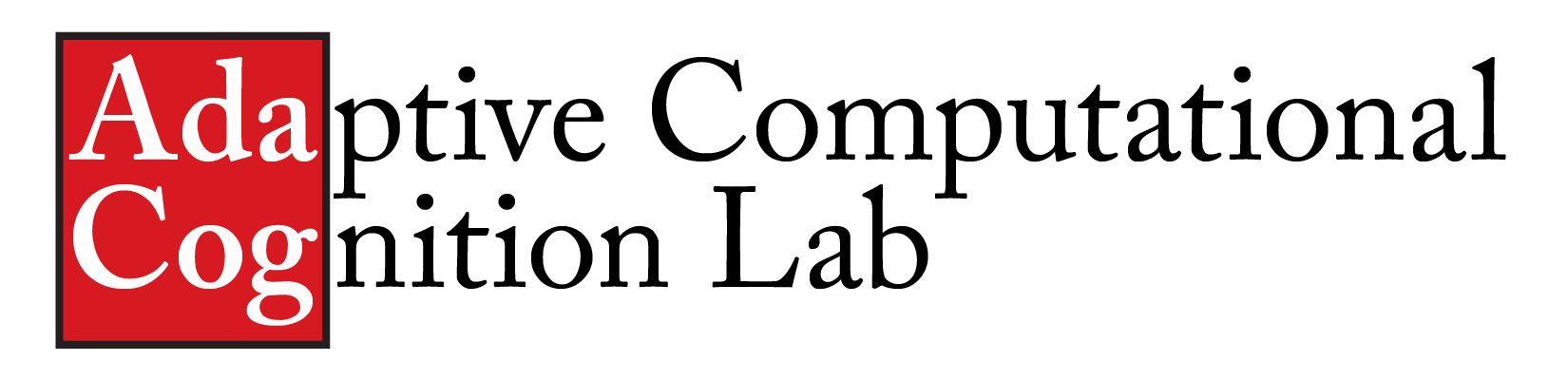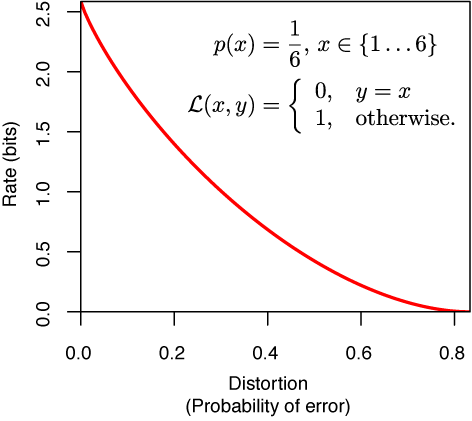Code for Rate-Distortion theoryI've written an R package for constructing models based on rate–distortion theory. The package is based on the material described in the paper:

Sims, C. R. (2016). Rate–distortion theory and human perception. Cognition, 152, 181-198.

Rate–distortion theory is a field within information theory that examines optimal lossy compression. That is, given that some information must be lost, how can a communication channel be designed that minimizes the cost of communication error? Rate-distortion theory is concerned with the optimal (minimal cost) solution to such tradeoffs. As such, the framework is particularly useful for constructing boundedly-rational models of biological information processing.

Installation instructions: The package is hosted on CRAN (the Comprehensive R Archive Network; https://cran.r-project.org/). To install the latest version, simply start R and enter the following command:

install.packages("RateDistortion")

Usage: The R package contains documentation on all the functions and includes minimal working examples. To get started, after installing within R, type:

library("RateDistortion")
?RateDistortion

As a simple example, consider a channel that transmits samples from a Gaussian distribution, minimizing the squared error cost function subject to a capacity constraint. This channel can be constructed as follows:

# Define a discretized Gaussian information source
x <- seq(from = -10, to = 10, length.out = 100)
Px <- dnorm(x, mean = 0, sd = 3)
Px <- Px / sum(Px) # Ensure that probability sums to 1
y <- x # The destination alphabet is the same as the source

# Define a quadratic cost function
cost.function <- function(x, y) {
(y - x)^2
}

R.max <- 2 # Assume a constraint on information rate (bits)
# Find an optimal information channel
Q <- FindOptimalChannel(x, Px, y, cost.function, R.max)

# Compute the conditional distribution for a given value of x
cpd <- ConditionalDistribution(Q, index = 25)

# Plot the results
plot(c(-10, 10), c(0, 0.15), type = "n",
xlab = "x", ylab = "Probability",
yaxs = "i")
lines(x, Px, col = "black") ## Prior distribution
abline(v = x, col = "red") ## Channel input
lines(cpd\$y, cpd\$p, col = "red") ## Channel output distribution

The manuscript linked above gives further examples on its use. Additional examples, along with more complete models and datasets, will eventually be posted here.